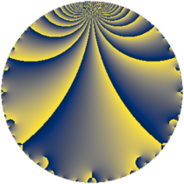Properties

 Label 405.4.bLevel $405$ Weight $4$ Character orbit 405.b Rep. character $\chi_{405}(244,\cdot)$ Character field $\Q$ Dimension $68$ Newform subspaces $6$ Sturm bound $216$ Trace bound $5$

Related objects

Defining parameters

 Level: $$N$$ $$=$$ $$405 = 3^{4} \cdot 5$$ Weight: $$k$$ $$=$$ $$4$$ Character orbit: $$[\chi]$$ $$=$$ 405.b (of order $$2$$ and degree $$1$$) Character conductor: $$\operatorname{cond}(\chi)$$ $$=$$ $$5$$ Character field: $$\Q$$ Newform subspaces: $$6$$ Sturm bound: $$216$$ Trace bound: $$5$$ Distinguishing $$T_p$$: $$2$$, $$11$$

Dimensions

The following table gives the dimensions of various subspaces of $$M_{4}(405, [\chi])$$.

Total New Old
Modular forms 174 76 98
Cusp forms 150 68 82
Eisenstein series 24 8 16

Trace form

 $$68 q - 252 q^{4} + O(q^{10})$$ $$68 q - 252 q^{4} - 56 q^{10} + 868 q^{16} - 8 q^{19} + 92 q^{25} + 76 q^{31} - 424 q^{34} + 344 q^{40} - 508 q^{46} - 1180 q^{49} + 1182 q^{55} + 1444 q^{61} - 3028 q^{64} + 1356 q^{70} - 2232 q^{76} + 1444 q^{79} - 410 q^{85} + 204 q^{91} - 6340 q^{94} + O(q^{100})$$

Decomposition of $$S_{4}^{\mathrm{new}}(405, [\chi])$$ into newform subspaces

Label Dim. $$A$$ Field CM Traces $q$-expansion
$a_{2}$ $a_{3}$ $a_{5}$ $a_{7}$
405.4.b.a $4$ $23.896$ $$\Q(\sqrt{3}, \sqrt{-17})$$ None $$0$$ $$0$$ $$0$$ $$0$$ $$q+(-2\beta _{1}-\beta _{2})q^{2}-9q^{4}+5\beta _{1}q^{5}+\cdots$$
405.4.b.b $8$ $23.896$ $$\mathbb{Q}[x]/(x^{8} + \cdots)$$ None $$0$$ $$0$$ $$-15$$ $$0$$ $$q+\beta _{1}q^{2}+(-3+\beta _{2})q^{4}+(-2+\beta _{4}+\cdots)q^{5}+\cdots$$
405.4.b.c $8$ $23.896$ $$\mathbb{Q}[x]/(x^{8} + \cdots)$$ None $$0$$ $$0$$ $$15$$ $$0$$ $$q+\beta _{1}q^{2}+(-3+\beta _{2})q^{4}+(2-\beta _{3})q^{5}+\cdots$$
405.4.b.d $16$ $23.896$ $$\mathbb{Q}[x]/(x^{16} + \cdots)$$ None $$0$$ $$0$$ $$0$$ $$0$$ $$q-\beta _{4}q^{2}+(-4-\beta _{1})q^{4}+(-\beta _{4}+\beta _{11}+\cdots)q^{5}+\cdots$$
405.4.b.e $16$ $23.896$ $$\mathbb{Q}[x]/(x^{16} + \cdots)$$ None $$0$$ $$0$$ $$-3$$ $$0$$ $$q+\beta _{1}q^{2}+(-3+\beta _{2})q^{4}+\beta _{9}q^{5}+(-\beta _{1}+\cdots)q^{7}+\cdots$$
405.4.b.f $16$ $23.896$ $$\mathbb{Q}[x]/(x^{16} + \cdots)$$ None $$0$$ $$0$$ $$3$$ $$0$$ $$q+\beta _{1}q^{2}+(-3+\beta _{2})q^{4}+\beta _{8}q^{5}+(\beta _{1}+\cdots)q^{7}+\cdots$$

Decomposition of $$S_{4}^{\mathrm{old}}(405, [\chi])$$ into lower level spaces

$$S_{4}^{\mathrm{old}}(405, [\chi]) \cong$$ $$S_{4}^{\mathrm{new}}(15, [\chi])$$$$^{\oplus 4}$$$$\oplus$$$$S_{4}^{\mathrm{new}}(45, [\chi])$$$$^{\oplus 3}$$$$\oplus$$$$S_{4}^{\mathrm{new}}(135, [\chi])$$$$^{\oplus 2}$$